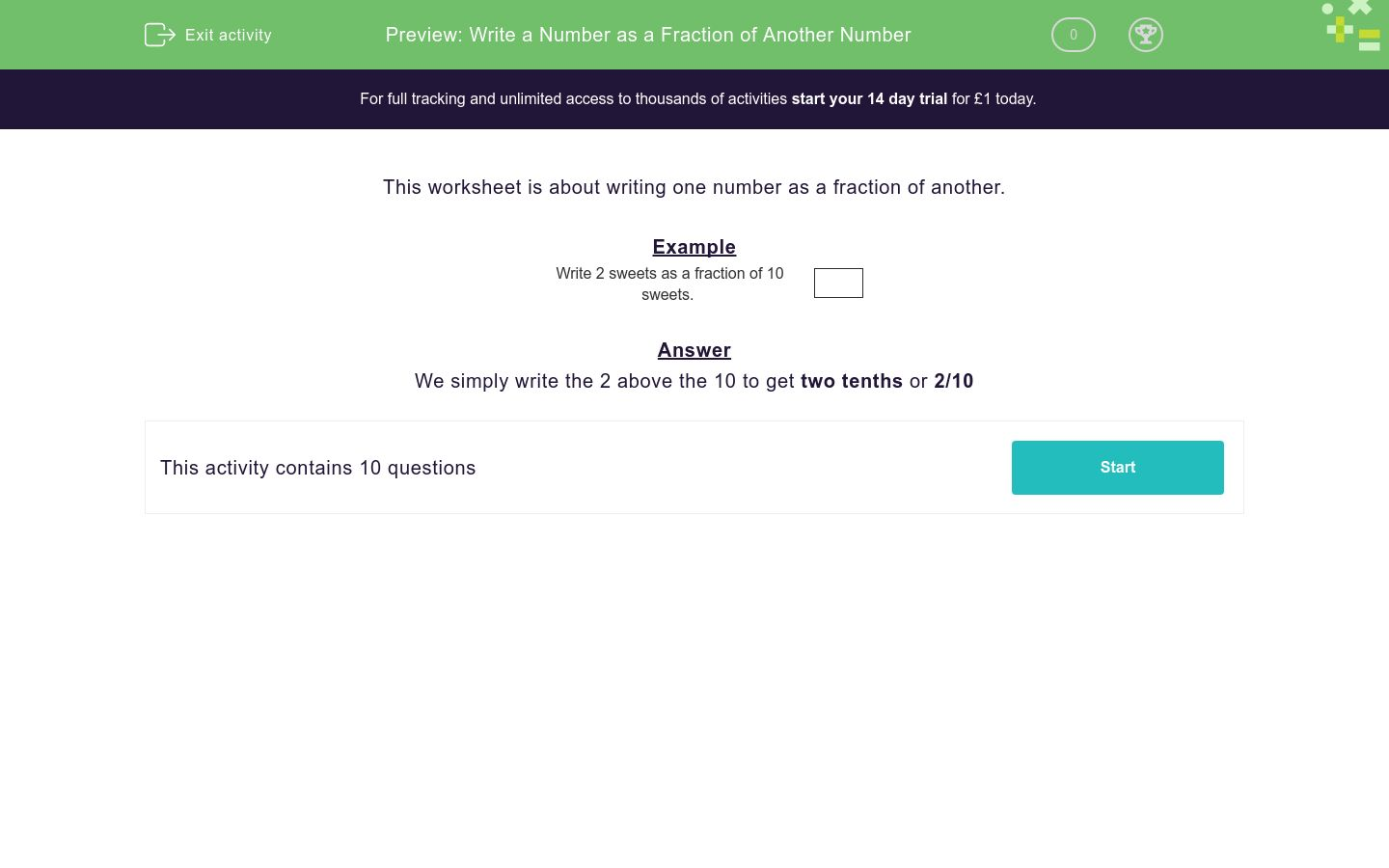# Write a Number as a Fraction of Another Number

In this worksheet, students write one number as a simple fraction of another without reducing the fraction.Key stage:  KS 2

Curriculum topic:   Number: Fractions and Decimals

Curriculum subtopic:   Solve Fraction Problems

Difficulty level:#### Worksheet Overview

This worksheet is about writing one number as a fraction of another.

Example

Write 2 sweets as a fraction of 10 sweets.